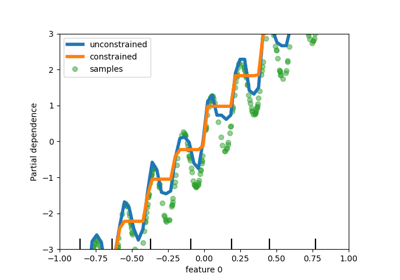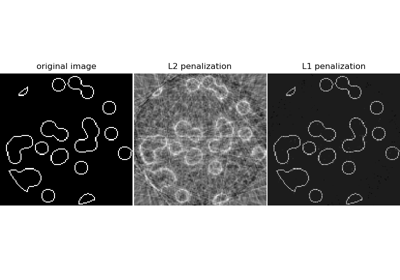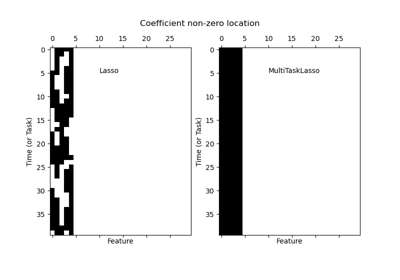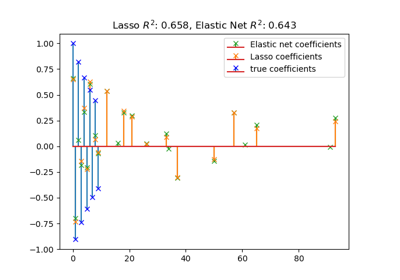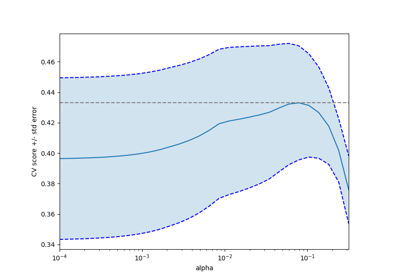# sklearn.linear_model.Lasso¶

class sklearn.linear_model.Lasso(alpha=1.0, *, fit_intercept=True, normalize=False, precompute=False, copy_X=True, max_iter=1000, tol=0.0001, warm_start=False, positive=False, random_state=None, selection='cyclic')[source]

Linear Model trained with L1 prior as regularizer (aka the Lasso)

The optimization objective for Lasso is:

(1 / (2 * n_samples)) * ||y - Xw||^2_2 + alpha * ||w||_1


Technically the Lasso model is optimizing the same objective function as the Elastic Net with l1_ratio=1.0 (no L2 penalty).

Read more in the User Guide.

Parameters
alphafloat, default=1.0

Constant that multiplies the L1 term. Defaults to 1.0. alpha = 0 is equivalent to an ordinary least square, solved by the LinearRegression object. For numerical reasons, using alpha = 0 with the Lasso object is not advised. Given this, you should use the LinearRegression object.

fit_interceptbool, default=True

Whether to calculate the intercept for this model. If set to False, no intercept will be used in calculations (i.e. data is expected to be centered).

normalizebool, default=False

This parameter is ignored when fit_intercept is set to False. If True, the regressors X will be normalized before regression by subtracting the mean and dividing by the l2-norm. If you wish to standardize, please use sklearn.preprocessing.StandardScaler before calling fit on an estimator with normalize=False.

precompute‘auto’, bool or array-like of shape (n_features, n_features), default=False

Whether to use a precomputed Gram matrix to speed up calculations. If set to 'auto' let us decide. The Gram matrix can also be passed as argument. For sparse input this option is always True to preserve sparsity.

copy_Xbool, default=True

If True, X will be copied; else, it may be overwritten.

max_iterint, default=1000

The maximum number of iterations

tolfloat, default=1e-4

The tolerance for the optimization: if the updates are smaller than tol, the optimization code checks the dual gap for optimality and continues until it is smaller than tol.

warm_startbool, default=False

When set to True, reuse the solution of the previous call to fit as initialization, otherwise, just erase the previous solution. See the Glossary.

positivebool, default=False

When set to True, forces the coefficients to be positive.

random_stateint, RandomState instance, default=None

The seed of the pseudo random number generator that selects a random feature to update. Used when selection == ‘random’. Pass an int for reproducible output across multiple function calls. See Glossary.

selection{‘cyclic’, ‘random’}, default=’cyclic’

If set to ‘random’, a random coefficient is updated every iteration rather than looping over features sequentially by default. This (setting to ‘random’) often leads to significantly faster convergence especially when tol is higher than 1e-4.

Attributes
coef_ndarray of shape (n_features,) or (n_targets, n_features)

parameter vector (w in the cost function formula)

sparse_coef_sparse matrix of shape (n_features, 1) or (n_targets, n_features)

sparse representation of the fitted coef_

intercept_float or ndarray of shape (n_targets,)

independent term in decision function.

n_iter_int or list of int

number of iterations run by the coordinate descent solver to reach the specified tolerance.

Notes

The algorithm used to fit the model is coordinate descent.

To avoid unnecessary memory duplication the X argument of the fit method should be directly passed as a Fortran-contiguous numpy array.

Examples

>>> from sklearn import linear_model
>>> clf = linear_model.Lasso(alpha=0.1)
>>> clf.fit([[0,0], [1, 1], [2, 2]], [0, 1, 2])
Lasso(alpha=0.1)
>>> print(clf.coef_)
[0.85 0.  ]
>>> print(clf.intercept_)
0.15...


Methods

 fit(X, y[, sample_weight, check_input]) Fit model with coordinate descent. get_params([deep]) Get parameters for this estimator. path(X, y, *[, l1_ratio, eps, n_alphas, …]) Compute elastic net path with coordinate descent. Predict using the linear model. score(X, y[, sample_weight]) Return the coefficient of determination R^2 of the prediction. set_params(**params) Set the parameters of this estimator.
__init__(alpha=1.0, *, fit_intercept=True, normalize=False, precompute=False, copy_X=True, max_iter=1000, tol=0.0001, warm_start=False, positive=False, random_state=None, selection='cyclic')[source]

Initialize self. See help(type(self)) for accurate signature.

fit(X, y, sample_weight=None, check_input=True)[source]

Fit model with coordinate descent.

Parameters
X{ndarray, sparse matrix} of (n_samples, n_features)

Data

y{ndarray, sparse matrix} of shape (n_samples,) or (n_samples, n_targets)

Target. Will be cast to X’s dtype if necessary

sample_weightfloat or array-like of shape (n_samples,), default=None

Sample weight.

check_inputbool, default=True

Allow to bypass several input checking. Don’t use this parameter unless you know what you do.

Notes

Coordinate descent is an algorithm that considers each column of data at a time hence it will automatically convert the X input as a Fortran-contiguous numpy array if necessary.

To avoid memory re-allocation it is advised to allocate the initial data in memory directly using that format.

get_params(deep=True)[source]

Get parameters for this estimator.

Parameters
deepbool, default=True

If True, will return the parameters for this estimator and contained subobjects that are estimators.

Returns
paramsmapping of string to any

Parameter names mapped to their values.

static path(X, y, *, l1_ratio=0.5, eps=0.001, n_alphas=100, alphas=None, precompute='auto', Xy=None, copy_X=True, coef_init=None, verbose=False, return_n_iter=False, positive=False, check_input=True, **params)[source]

Compute elastic net path with coordinate descent.

The elastic net optimization function varies for mono and multi-outputs.

1 / (2 * n_samples) * ||y - Xw||^2_2
+ alpha * l1_ratio * ||w||_1
+ 0.5 * alpha * (1 - l1_ratio) * ||w||^2_2


(1 / (2 * n_samples)) * ||Y - XW||^Fro_2
+ alpha * l1_ratio * ||W||_21
+ 0.5 * alpha * (1 - l1_ratio) * ||W||_Fro^2


Where:

||W||_21 = \sum_i \sqrt{\sum_j w_{ij}^2}


i.e. the sum of norm of each row.

Read more in the User Guide.

Parameters
X{array-like, sparse matrix} of shape (n_samples, n_features)

Training data. Pass directly as Fortran-contiguous data to avoid unnecessary memory duplication. If y is mono-output then X can be sparse.

y{array-like, sparse matrix} of shape (n_samples,) or (n_samples, n_outputs)

Target values.

l1_ratiofloat, default=0.5

Number between 0 and 1 passed to elastic net (scaling between l1 and l2 penalties). l1_ratio=1 corresponds to the Lasso.

epsfloat, default=1e-3

Length of the path. eps=1e-3 means that alpha_min / alpha_max = 1e-3.

n_alphasint, default=100

Number of alphas along the regularization path.

alphasndarray, default=None

List of alphas where to compute the models. If None alphas are set automatically.

precompute‘auto’, bool or array-like of shape (n_features, n_features), default=’auto’

Whether to use a precomputed Gram matrix to speed up calculations. If set to 'auto' let us decide. The Gram matrix can also be passed as argument.

Xyarray-like of shape (n_features,) or (n_features, n_outputs), default=None

Xy = np.dot(X.T, y) that can be precomputed. It is useful only when the Gram matrix is precomputed.

copy_Xbool, default=True

If True, X will be copied; else, it may be overwritten.

coef_initndarray of shape (n_features, ), default=None

The initial values of the coefficients.

verbosebool or int, default=False

Amount of verbosity.

return_n_iterbool, default=False

Whether to return the number of iterations or not.

positivebool, default=False

If set to True, forces coefficients to be positive. (Only allowed when y.ndim == 1).

check_inputbool, default=True

Skip input validation checks, including the Gram matrix when provided assuming there are handled by the caller when check_input=False.

**paramskwargs

Keyword arguments passed to the coordinate descent solver.

Returns
alphasndarray of shape (n_alphas,)

The alphas along the path where models are computed.

coefsndarray of shape (n_features, n_alphas) or (n_outputs, n_features, n_alphas)

Coefficients along the path.

dual_gapsndarray of shape (n_alphas,)

The dual gaps at the end of the optimization for each alpha.

n_iterslist of int

The number of iterations taken by the coordinate descent optimizer to reach the specified tolerance for each alpha. (Is returned when return_n_iter is set to True).

Notes

For an example, see examples/linear_model/plot_lasso_coordinate_descent_path.py.

predict(X)[source]

Predict using the linear model.

Parameters
Xarray_like or sparse matrix, shape (n_samples, n_features)

Samples.

Returns
Carray, shape (n_samples,)

Returns predicted values.

score(X, y, sample_weight=None)[source]

Return the coefficient of determination R^2 of the prediction.

The coefficient R^2 is defined as (1 - u/v), where u is the residual sum of squares ((y_true - y_pred) ** 2).sum() and v is the total sum of squares ((y_true - y_true.mean()) ** 2).sum(). The best possible score is 1.0 and it can be negative (because the model can be arbitrarily worse). A constant model that always predicts the expected value of y, disregarding the input features, would get a R^2 score of 0.0.

Parameters
Xarray-like of shape (n_samples, n_features)

Test samples. For some estimators this may be a precomputed kernel matrix or a list of generic objects instead, shape = (n_samples, n_samples_fitted), where n_samples_fitted is the number of samples used in the fitting for the estimator.

yarray-like of shape (n_samples,) or (n_samples, n_outputs)

True values for X.

sample_weightarray-like of shape (n_samples,), default=None

Sample weights.

Returns
scorefloat

R^2 of self.predict(X) wrt. y.

Notes

The R2 score used when calling score on a regressor uses multioutput='uniform_average' from version 0.23 to keep consistent with default value of r2_score. This influences the score method of all the multioutput regressors (except for MultiOutputRegressor).

set_params(**params)[source]

Set the parameters of this estimator.

The method works on simple estimators as well as on nested objects (such as pipelines). The latter have parameters of the form <component>__<parameter> so that it’s possible to update each component of a nested object.

Parameters
**paramsdict

Estimator parameters.

Returns
selfobject

Estimator instance.

property sparse_coef_

sparse representation of the fitted coef_

## Examples using sklearn.linear_model.Lasso¶